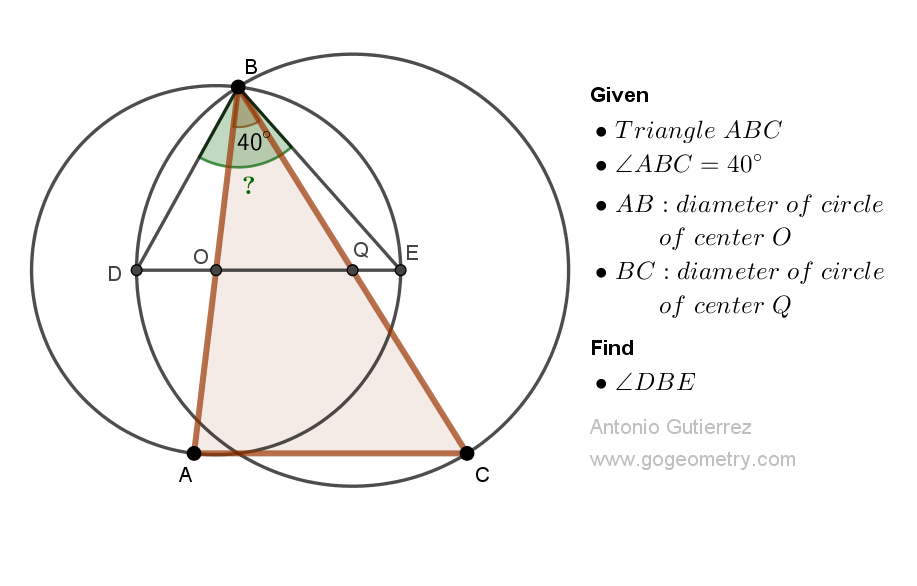## Monday, March 6, 2023

### Geometry Problem 1521: Unlock the Secret to Finding the Measure of an Angle in a Triangle with Two Sides as Diameters of Circles. Difficulty Level: High School.

Geometry Problem 1521. Post your solution in the comment box below.
Level: Mathematics Education, K-12 School, Honors Geometry, College.

Details: Click on the figure below.More Details

To post a solution to this problem click Comment underneath the post, or click into the line that says, “Enter Comment.” Type what you want to say and press Publish to post your solution.

1.DBE = 70° (It will be explained on the sketch)

2.Let <QBD = < QDB = p and let < OBE = < OEB = q

In Triangle OQB,
(p+p-40) + (q + q - 40) = 140
So p + q = 220/2 = 110

Hence < DBE = p + q - 40 = 70

Sumith Peiris
Moratuwa
Sri Lanka